[BACK]
 Computer Systems Science & EngineeringDOI:10.32604/csse.2023.024049Article

Design of Clustering Techniques in Cognitive Radio Sensor Networks

1Department of Electronics and Communication Engineering, SRM TRP Engineering College, 621105, Tamil Nadu, India
2Department of Computer Science and Engineering, Sriram Engineering College, 602024, Tamil Nadu, India
3Department of Electronics and Communication Engineering, Saveetha School of Engineering, 602105, Tamil Nadu, India
4Department of Electronics and Communication Engineering, Vishnu Institute of Technology, Bhimavaram, 534202, Andhra Pradesh, India
*Corresponding Author: R. Ganesh Babu. Email: ganeshbaburajendran@gmail.com
Received: 01 October 2021; Accepted: 05 January 2022

Abstract: In recent decades, several optimization algorithms have been developed for selecting the most energy efficient clusters in order to save power during transmission to a shorter distance while restricting the Primary Users (PUs) interference. The Cognitive Radio (CR) system is based on the Adaptive Swarm Distributed Intelligent based Clustering algorithm (ASDIC) that shows better spectrum sensing among group of multiusers in terms of sensing error, power saving, and convergence time. In this research paper, the proposed ASDIC algorithm develops better energy efficient distributed cluster based sensing with the optimal number of clusters on their connectivity. In this research, multiple random Secondary Users (SUs), and PUs are considered for implementation. Hence, the proposed ASDIC algorithm improved the convergence speed by combining the multi-users clustered communication compared to the existing optimization algorithms. Experimental results showed that the proposed ASDIC algorithm reduced the node power of 9.646% compared to the existing algorithms. Similarly, ASDIC algorithm reduced 24.23% of SUs average node power compared to the existing algorithms. Probability of detection is higher by reducing the Signal-to-Noise Ratio (SNR) to 2 dB values. The proposed ASDIC delivers low false alarm rate compared to other existing optimization algorithms in the primary detection. Simulation results showed that the proposed ASDIC algorithm effectively solves the multimodal optimization problems and maximizes the performance of network capacity.

Keywords: Adaptive swarm distributed clustering; cognitive radio; clustering algorithm; distributed swarm intelligent; energy efficient distributed cluster-based sensing; multi modal optimization

1  Introduction

In the modern communication scenarios, spectrum sensing more accurately in Cognitive Radio (CR) is an important task . For identifying the best spectrum nodes, several algorithms have been proposed. Among all the secondary users, co-operative sensing collects precise sensing information with maximum system reliability and shorten sensing time . The sensed information are transmitted from the secondary users without any centralized control unit . All the cognitive users in the distributed co-operative sensing find the un-used primary channel, where it reduces the time delay and transmission burden in order to take decisions on the presence of the primary signal . In recent decades, several clustering algorithms like Groupwise Constrained Agglomerative, k-means, Distributed Spectrum Aware (DSAC), and k-neighbourhood clustering processes have been developed [5,6]. The clustering process is often fine tuned by using optimization algorithms, such as: Jumper Firefly Algorithm (JFA), Particle Swarm Optimization (PSO), and Firefly Algorithm (FA), just to mention a few. The energy consumption will be high, while a sink station from CH is at far distance. In order to address the above stated concern, PSO algorithm is used in this protocol for improving the life-time and the energy efficiency. The optimization algorithms create clusters and the cluster head is detected based on the energy. Then, data transmission starts for the sensor nodes using the shortest path. Wang et al.  developed a firefly algorithm for node clustering, which includes two types of clustering: partitional clustering and hierarchical clustering. The hierarchical approach groups many hierarchical clusters into a small number of clusters with nearby centroids. It can be implemented in two different ways: (i) the agglomerative method iteratively merges the two more similar clusters until one big cluster, containing all data, is obtained; (ii) the divisive method iteratively divides the most dissimilar groups, until each object belongs to a single cluster. The partitional clustering creates disjoint clusters of data, without creating a hierarchical structure. Data in each clusters are represented by prototypes. In the reference , the authors used JFA in the BS to identify the optimal solution in the status table. The status of the table is observed which provides a suitable scenario based function for the jumping process. The process is executed on the search agents in order to make the decision process.

The present research work proposes a new clustering algorithm that has been developed to increase the life-time of CR sensor networks with clusters that are energy efficient. The proposed research work focused on the co-operative sensing between the secondary users. The main aim of this research paper is to collect the precise sensing information in order to decrease the number of false alarms, to improve the system reliability which shortens the sensing time and increases the detection rate. Finally, heuristic algorithms, such as: Distributed Swarm Optimized (DSO), Distributed Jumper Firefly Optimized (DJFO), Distributed Firefly Optimized (DFO), and Distributed Swarm Intelligent Based (DSIB) clustering techniques that have been used for comparison.

In the Distributed Swarm Optimized clustering (DSOC) algorithm, each cluster node is moved towards the best swarm particle with minimum neighbourhood distance . Then, every particle velocity and position are evaluated on the basis of the objective function, where the stop criterium is the global best position. Results have shown that the convergence rate of the DSOC algorithm is comparable to the genetic algorithms it is compared to. However, DSOC algorithm suffers of slow convergence rate and weak local search ability.

In order to overcome these limits, Distributed Firefly Optimized clustering algorithm (DFOC) has been developed, and it is able to group the best cognitive nodes . In DFOC algorithm, all the cognitive nodes are moved towards the brighter firefly in order to generate an organized cluster with minimum computational time. At the critical positions, the fireflies are disappeared in the DFOC algorithm, and it is not able to memorize the history of the past events while doing the clustering without status table. Thus, a third algorithm, namely Distributed Jumper Firefly Optimized DJFO clustering (DJFOC) has been proposed in . The DJFOC and DFOC are non-linear optimization algorithms, that are based on firefly attractiveness and their intensity behavior. The DJFOC algorithm is utilized for collecting an entire present records situation and changes are stored in the status of table. The DJFOC algorithm improves the spectrum access of SUs and PUs more than what DFOC is able to do. Convergence speed of DJFOC algorithm is higher, if compared to the DFOC, and DSOC algorithms. The DJFOC algorithm obtained high probability of detection and optimal number of cluster communication.

Finally, the optimization algorithm Adaptive Swarm Distributed Intelligent based Clustering algorithm (ASDIC) mainly aimed at reducing the average sensing time of the PUs by using cooperative SUs, since it cannot be parallelized with all the positions. By utilizing the objective functions, the intensity of light force is calculated and the whole population is partitioned into sub-swarms. In this research, the performance analysis is investigated for several clustering algorithms like DFOC, DSOC, DJFOC, and ASDIC.

The below mentioned performance measures are taken into consideration for comparison:

• Conservative Merge Duration with CRSN Sizes;

• Conservative power of nodes for respective cluster numbers;

• Conservative power for PU’s and SU’s nodes;

• Spectrum sensing detection scheme.

This paper is organized as follows: Section 2 addresses the existing research works in cognitive radio networks along with its issues. A summary of the proposed ASDIC based ASDIC algorithm and cluster communication power reduction is given in Section 3. Results and discussion of the proposed algorithm are detailed in Section 4. Finally, conclusions are derived in Section 5 together with future work directions.

2  Background

Rahim et al.  presented Gale–Shapley matching algorithm to develop channel allocation approach for the SUs of a Cognitive Radio Network (CRN). The channel allocation approach called Secondary Proposed Deferred Acceptance (SPDA) was used to discover an adequate channel for SUs from the available channels. Here, the channel allocation was carried out by considering two different parameters such as SU class of service requirements and channel quality. Therefore, the developed SDPA was used to increase the throughput at secondary network with less interference to the primary network. However, the developed SPDA considered only the SU satisfaction, does not take into account neither the PU or SU satisfaction.

Han et al.  analyzed the average Spectrum Efficiency (SE) for Multi-Radio Multi-Hop (MRMH) CRN using the information of multi-slot statistical spectrum status. Next, the Multi-step Convex Approximation (SMCA) scheme was developed for converting the non-convex complex fractional Mixed Integer Nonlinear Programming (MINLP) characteristic into successive convex one using ε iteration convex optimization method. However, the SMCA used in the CRN has little bit higher complexity, during the data transmission.

Shakeel et al.  presented Markov-based analytical model determined the gain for non-switching spectrum handoff technique with respect to multi-class SUs. The hybrid interweaves with spectrum access in CRN access the model approach to underlay and interweave spectrum access methods. In a multiclass SUs, the prioritized class is considered in the traffic for accessing the hybrid spectrum access method satisfied the Quality of Service (QoS) in the delay sensitive traffic. If the CRN has higher primary traffic load, the SU was required to be in a waiting status more time for each channel before becoming free.

Fang et al.  developed Semi-Tensor Product Compressed Spectrum Sensing (STP-CSS) using semi-tensor product for obtaining the reconstruction of spectral signals and energy compression. A group of wideband random filters was used to develop STP-CSS method. Subsequently, the spectrum sensing’s speed was increased by accomplishing the perceptual reconstruction process in parallel. However, the energy consumption of the CRN was increased, while decreasing the compression ratio.

Mi et al.  presented semi-soft decision scheme for the Cooperative Spectrum Sensing (CSS), which satisfies the tradeoff among the band cost and sensing performance. The sensing approach was constructed using three modules. At first, the energy detection based PU signal detection was obtained using a local detection module and the decision results are transmitted as 1-bit or 2-bit information. Next, the collected decision information was evaluated by Fusion Center (FC) over a data reconstruction module. Further, the final decision was made by fusing the evaluated data at the global decision module. However, the CRN required higher bandwidth, while enormous amount of SU send the data to FC.

All these previous research papers have various limitations; higher overhead, high energy consumption, high latency, and an inappropriate satisfaction between the SU and PU. In order to overcome the aforementioned limitations, the ASDIC is developed in the network to increase the convergence speed. Additionally, the ASDIC is useful to increase the detection performances.

3  Proposed Adaptive Swarm Distributed Intelligent Based Clustering Algorithm For Cognitive Radio Networks

In Fig. 1, the proposed ASDIC technique is introduced. In the dynamic spectrum environment, the CRSNs are divided based on the connectivity of the clustered structures represented as ‘K’ and each of the CRSN nodes discovers the channels. The PUs and SUs operate for specific bands of frequency and are assigned consecutively and continuously. Therefore, re-clustering process problem occurred in the existing methods are overcome in the Cognitive Radio Sensor Nodes (CRSN) and nodes will be grouped based on the swarm intelligence that is embedded in the clustering technique. There are various number of nodes present in the sensor network and an optimal cluster are formed from those nodes. The optimal clusters are found using the following equation 

Kopt=[Ndmax3ρ+0.5] (1)Figure 1: An illustration for cooperative ASDIC clustering structure

From the equation N is known as the nodes that are available totally, ρ is known as CRSN totally available corresponding to the unit area, and maximal transmission range among the CRSN nodes is represented as dmax.

The CH is selected among the number of clusters in the BS communication range randomly. The list of CHs selected sends the information from the BS to the respective positions for the leftover CRSN nodes. Each of the CRSN nodes functions are used to determine the distance between themselves and the CHs nodes. The proposed ASDIC ranks the CHs based on the least cost fitness function which obtains the best cost fitness function among the presented CRSN nodes and has less converging time for CRSN size having a large size. The lifetime of the CRSN energy is increased for efficient clusters that form the dynamic channel allocation. When the SUs have placed the channels, they are not accessible among the PUs transmission range. The spectrum opportunistically tries to access the dynamic spectrum methods that are used for next-generation networks. The locally sensed information is shared with each SUs to other SUs without centralized control unit. All the cognitive users preset finds the primary unused channel in the distributed cooperative sensing environment accordingly.

Based on the best spectrum band available utilized the CR for communication. The characteristics of the spectrum, reconfiguration functions of CR, and the user requirement selection are decided by Spectrum management. The finest suitable spectral band from the xG network is adopted to improve the overall requirements in terms of the overall QoS spectrum. The channels are detected in the available either are detected by SUs or PUs avoids the interferences. The PU corresponds to distinct channels which are represented with their respective colors. The common channel is not conquered by PU nodes that are available in CRSN nodes or in the same cluster. Each CHs sense the channels that are available and selects one channel that assigns one among all their presented channel members. At the time of channel selection, the condition checks the cluster head that selects the channel using the nearest PUs. The best CHs are communicated with different clustering and their channel members are allocated based on the least clustering node power that solves the multimodal optimization problems. The hypothesis model for detecting the PUs checks using the following condition.

r(t)={n(t)H0hs(t)+n(t)H1 (2)

From the equation r(t) indicates the received signal from SUs. s(t) is the signal transmitted from PUs, n (t) is the zero mean of AWGN. ‘h’ is the channel’s amplitude gain. ‘H0’ represents the absence of PU over the certain spectrum band channel which is also known as ‘null hypothesis’. Similarly, ‘H1’ is known as the PU presence over the channel which is also referred to as the ‘presence hypothesis’.

3.1 Calculations of Power Using ASDIC Algorithm

The CRSN nodes are connected with each CH for computing the minimum distance with the BS and for selecting the CH. The cluster has to follow these two conditions:

• The communication range of BS should be inside the CH.

• The high residual energy should be possessed by CH.

The total transmission power used for communication which is given by:

Ptx=k=1Ki=1NDistmin(nik,Centerk(CH)) (3)

The minimum distance between the two points of pair of coordinates such as (x0,y0) and (x1,y1) is calculated by using the following:

Distmin=(x0x1)2+(y0y1)2 (4)

The intra cluster communication between the Cluster Members (CMs) have the ability for sending the information from the source node to that of the CH center position for all the available channel based on the shortest distance for node communication. The minimum distance for the k-th cluster with i-th node is represented as ‘ ck ’ and the center position of CH for kth cluster is represented by

Pintra(Tot)=k=1KDistmin(nik,Center(CH))Ck

=k=1KDistmin(nik,njk)ck (5)

Similarly, in the inter cluster communication, the CH position is at the center gathers the information from the source node compressively communicates with the CHs that are nearer finds the shortest communicating power among the clustered centers. The minimum distance for the k-th cluster with j-th node is represented as ‘ ck ’ and CH central position for kth cluster is indicated by ( ck ) is represented as

Pinter(Tot)=k=1K(i=1Nj=1MDistmin(Centerk(CHi),Centerk(CHj)))ck

=k=1K(ijDistmin(Centerk(CHi),Centerk(CHj)))ck (6)

where Centerk(CHi) is the i-th CH’s center position in k-th cluster ( ck ) and Centerk(CHj) is the j-th cluster center position for the k-th cluster is ck .

The coordinates are used for calculating the minimum distance which is expressed as shown in the below equation. P(Tot) is represented as the summation between the power for inter clustering and intra clustering communication. where, Pinter is the power for inter clustering communication and Pintra is the power obtained for clustering communication.

P(Tot)=i=1N(Pintra+Pinter)ni (7)

3.2 Proposed ASDIC Algorithm

From the Fig. 2, the proposed ASDIC sensor network consists of ‘N’ number of nodes and predetermined clusters of ‘N’ are represented as ‘K’ is composed of following:

1. ‘S’ is the number of elements sets for comprising ‘K’ CHs chosen arbitrarily among all the CH suitable candidates. At each node point ni (i = 1,2,..,N) determines the distance d(ni, CHp,k) among the node of all CHs positioned points allocates the CH point at each node ni. Here, CHp,k is the k-th CH of the particle p, d(ni, CHp,k) = min{d(ni, CHp,k)} for k = 1,2,.., K.

2. The cost function estimation for each arbitrary is chosen by CH calculates the best CH for transmission using FA. The rules for clustering process is followed for acknowledging the BS using the centralized algorithm . The BS runs FA finalizes the CHs to find the best optimal position for cost function.

Cost=f1×β+f2×(1β) (8)

f1=maxk=1,2,...K{niCp,kd(ni,CHp,k)/|Cp,k| (9)

f2=i=1NE(ni)k=1KE(CHp,k) (10)

where β is known as the user defined constant. β=0.5 , the CHs are associated with K nodes for the cluster particle p gives the maximum average distance associated is represented as f1. The function f2 is the ratio for the average energy for the nodes of CHs. E(ni) is known as the energy for i-th node and the energy of the kth node for particle p is represented as E(CHp,k).

3. The CHs chooses the available channels in their range.

4. The channel having the high quality is selected based on the condition selects the channel by using the nearby PUs.

5. The data from the cluster members of CHs are aggregated though the available local common channel.

6. Lastly from the BS the collected information is transmitted to CHs.Figure 2: ASDIC flow chart

4  Simulation Results and Discussion

This section describes evaluation of performance for the proposed DJFOC using Network Simulator-2 (NS2) simulation for the CR network . The Fig. 3 shows total number of PUs and SUs for accessing the channels based on the optimal ‘K’ number of clustered structure connectivity. The 10 PUs and 90 SU nodes are utilized during the simulation to evaluate the performance of dynamic spectrum access of the proposed model. The user nodes are randomly placed on the 1000 × 1000 m fields and the channels available are marked as 10 colors such as pink, deep pink, blue cyan, sky blue, yellow-green, maroon, yellow, violet red and green colored selections. The representation for the channels occupied by SUs are C0, C1,…,C9 and for PUs are from 0,1,…,9 in NS2 simulation (Ver. 2.34). Each PU selects the common 10 channels and the range of fortification is about 200 m where the CRSN neighbour remained is not possible for accessing the channels already occupied. The analysis is performed out from the simulation time of 131 s and packet size is constant of about 512 byte.Figure 3: The process of Channel distribution for SUs and PUs

In this section execution correlation is investigated for the existing DGCC strategy with different proposed advanced grouping strategies by utilizing the measurements of Conservative merge time for different CRSN sizes, Conservative node power for various group numbers, Conservative node power for PU’s and SU’s, detection probability and missed recognition with different estimations of PFA, detection probability with different estimations of SNR. Fig. 4 shows the general examinations of Conservative Merge Duration for Various CRSN Sizes in various improved grouping strategies. From the Fig. 4, it is seen that Conservative unite time for proposed ASDIC is less contrasted with other enhanced grouping strategies. The proposed ASDIC Conservative combine time is 47.2 s lesser than existing DGCC for different CRSN sizes.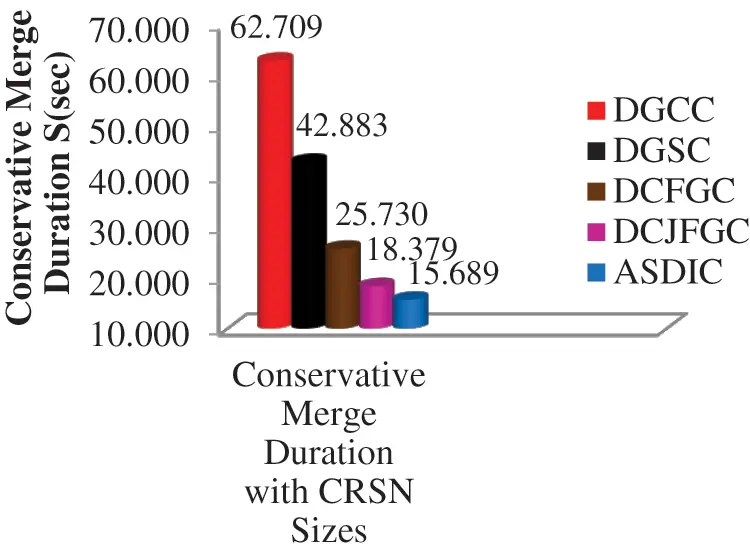Figure 4: Correlation of conservative merge duration in various improved grouping strategies

From the Fig. 5, it is noticed that Conservative node power for proposed ASDIC is less contrasted with other advanced grouping strategies. The proposed ASDIC Conservative node power is 2585.774 μW lesser than existing DGCC for different cluster number. Essentially, it is noticed that Conservative node power of main and secondary users for proposed ASDIC is less contrasted with other improved grouping strategies. The ASDIC Conservative node power of primary user is 75.3 μW lesser than DGCC. The ASDIC Conservative node power of the secondary user is 281.978 μW lesser than DGCC. Fig. 5 shows the general examinations of Conservative Node Power for Various Cluster Numbers, PU’s and SU’s in various improved grouping strategies.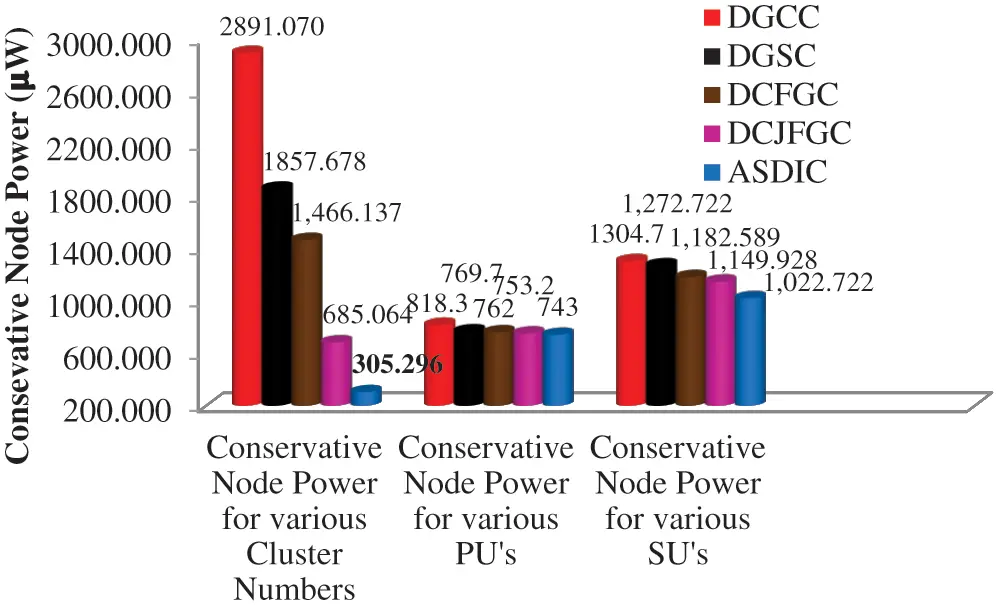Figure 5: Correlation of conservative node power in various improved grouping strategies

The proposed scheme is used for analyzing the convergence time (Average) for distinct sizes of CRSN with the average node power for PUs and SUs. The average power of the node for different cluster numbers is used for performance detection. The size of CRSN plays a significant role for interacting with the BS. The main objective is to select the best CHs among the effective multiple users in grouped clusters that reduce the converging time, power of nodes, and interference. The average convergence time for ASDIC is lesser as compared with the existing optimizing clustering techniques. The proposed ASDIC showed an average convergence time of 47.2 s lesser when compared with the existing DSAC method having distinct CRSN size obtained the performance measure as 74.98%.

Fig. 6 describes the comparative analysis for an average converge time for different CRSN size with the various optimized clustering approach. When the size of CRSN in-crease, the average convergence time maximizes linearly. The convergence time is required to evaluate the speed factors proposed ASDIC method. The maximum of 280 is CRSN size considered in this work.

From Fig. 6 it was examined that the proposed ASDIC obtained power for average node lesser when compared to the existing methods of clustering. An average node power of 2585.774 μW is obtained for the proposed ASDIC which is lesser than the existing DSAC for many number of clusters obtained performance as 89.44%. The Fig. 7 shows the comparative graph for the power for average nodes with respect to many number of clusters as compared with the various optimized clustering approach. The graph describes the power observed from many number of clusters as 2 to 28. For an average node the power for CRSN is the ratio of the overall energy of SUs and PUs with the overall number of nodes in the clusters that is expressed in watt (W).

AverageNodePower=i=1NPUi(Energy)+i=1NSUi(Energy)TotalNumberofnodesinclusters (11)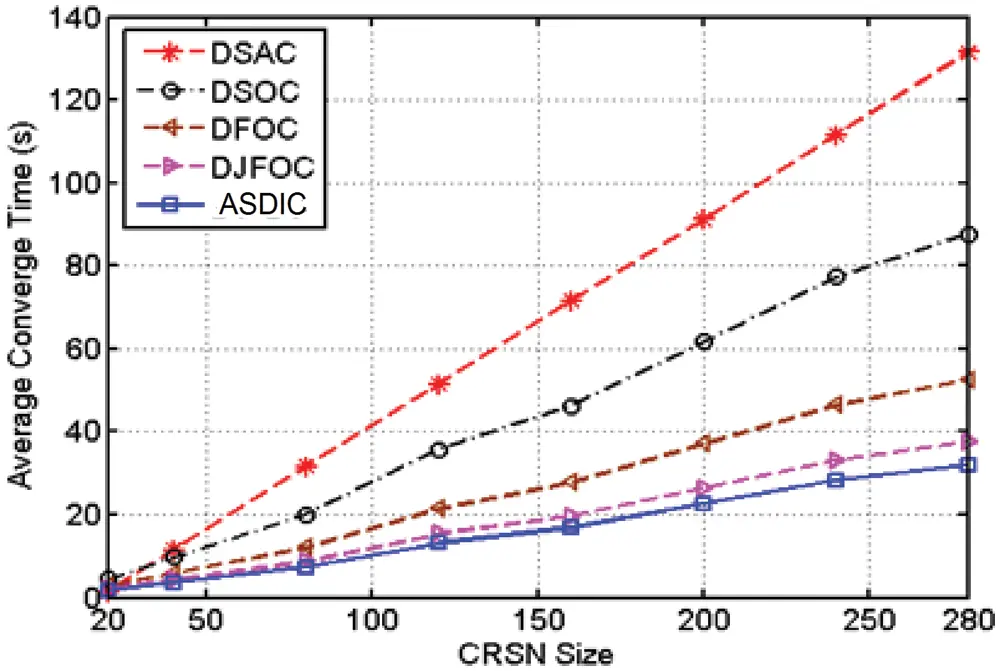Figure 6: The comparison graph of average convergence time for different CRSN size with the existing optimized clustering approaches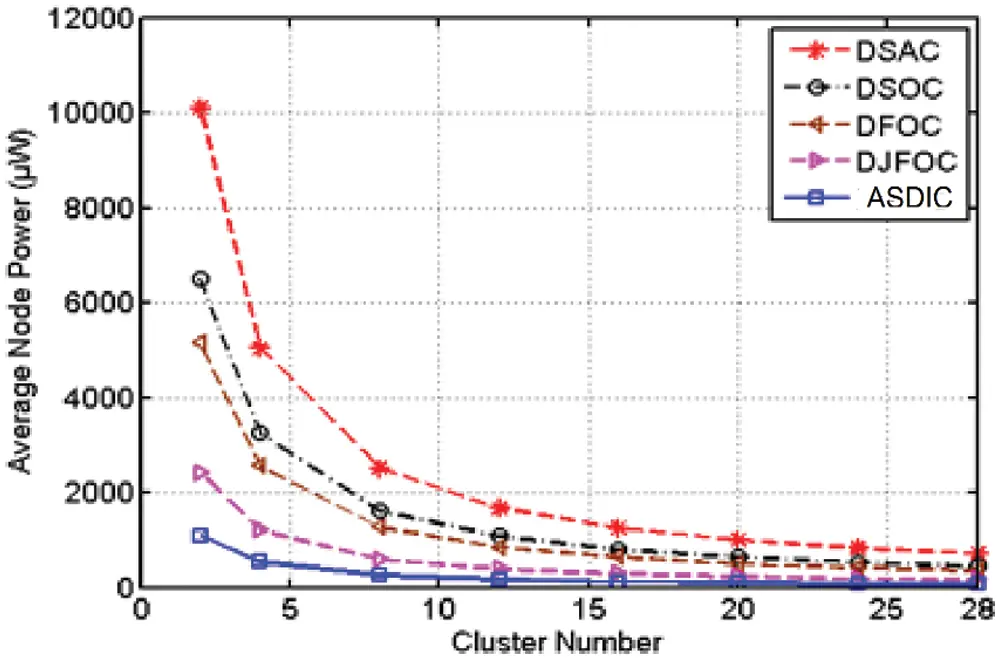Figure 7: The comparison graph of average nodes power for different cluster numbers with the existing optimized clustering approaches

Fig. 7 describes that the power remains same if the dimension of clusters is higher than 28, that time simulation will be ended. Fig. 7 shows cluster number against the average node power values for the existing DFOC, DSAC, DSOC, DJFOC, and DSIBC techniques. During the analysis combining the PUs with SUs in the network indicates the lower and higher power at the nodes using the ASDIC algorithm. The PU’s average node power is the ratio of overall energy of PUs to overall number of Pus that is expressed in watt (W).

AverageNodePowerforPUs=i=1NPUi(Energy)TotalNumberofPUs (12)

Average power increases linearly with the number of PUs. The Fig. 8 describes the values of PU’s number verses average node power with existing optimized clustering approaches for 10 PU’s node. The average node power of ASDIC is 75.3 μW for primary user which is lesser than the DSAC with the percentage of “9.646%”.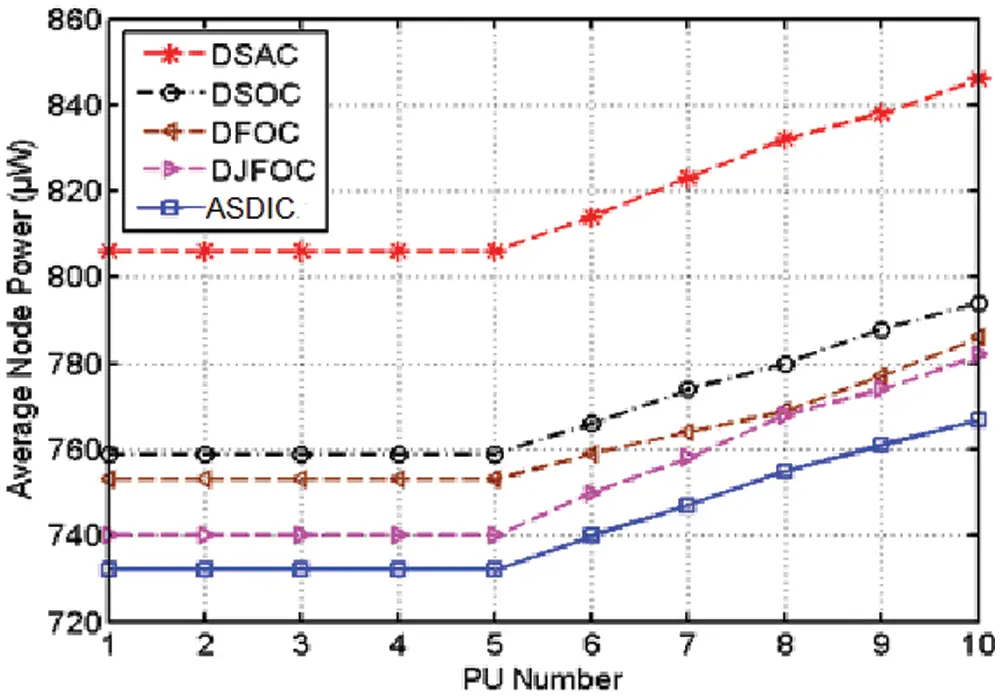Figure 8: The average node power for PU’s with existing optimized clustering approaches

Fig. 8 describes the comparisons of average node power in PUs with existing optimized clustering approaches. When more number of PUs are in the range of transmission, the process of clustering needs more spectrum resources. So, the results of clustering are affected by energy consumption. Thus, 10 nodes of primary user are considered with 200 m of fortification range during the simulation. The ASDIC is effective among existing optimized clustering approaches by enhancing utilization of spectrum within the PU’s for lesser power of average node.

Similarly, an average node power of SUs are estimated by using equation.

AverageNodePowerforSUs=i=1NSUi(Energy)TotalNumberofSUs (13)

The number of SUs will increase linearly as the node power also increases. Fig. 9 shows the results obtained for the optimized clustering process for node 90 SU. The node power averagely for ASDIC for the secondary user is obtained as 281.978 μW shows lesser percentage when compared to the existing DSA for SUs average node power. The comparison for an average node in terms of power for distinct SUs with the existing optimized clustering techniques. During simulation, the 90 nodes of secondary user are utilized within 150 m of fortification range. The proposed ASDIC approach is effective than the existing optimized clustering approaches by enhancing the utilization spectrum with the minimum average node power of secondary users.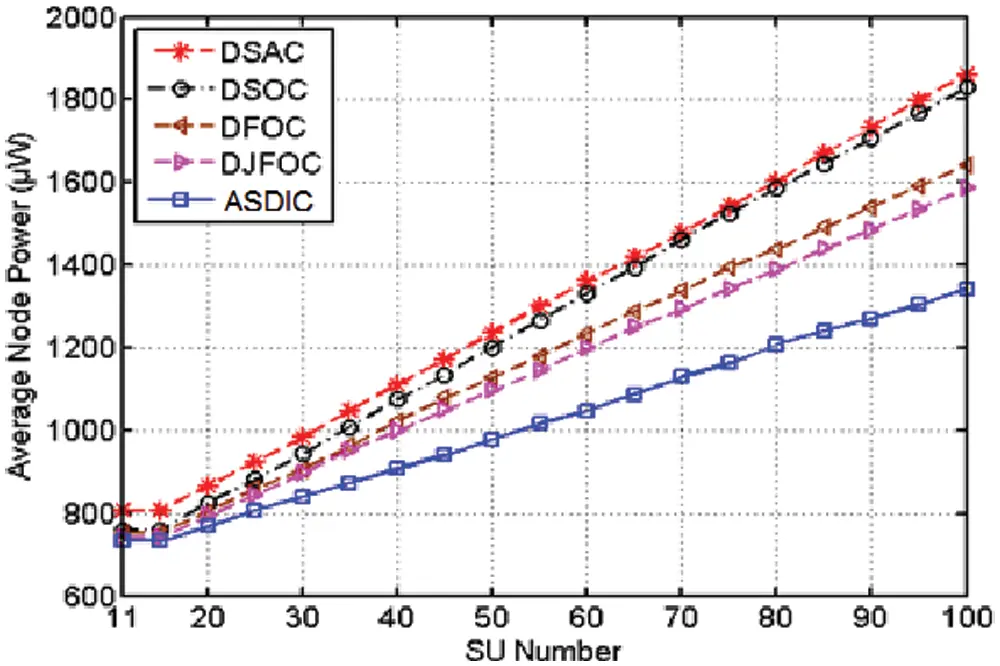Figure 9: The comparison graph of SUs average node power with existing optimized clustering approaches

The singular threshold detectors perform better in the co-operative spectrum of sensing network by higher detection rate with lesser false rates. During the stage of detection, the sensing noise or error in the cooperative node over the channel is deleted with the reliable decision. The performance of detection for spectrum sensing approach will be evaluated by utilizing the false alarm rate, missed detection and detection . The estimate of SNR for detecting the received signal and to determine the output from performance of detection with spectrum sensing approaches.

The threshold value of λ = 4 dB is on the basis of observations and experimental results .

a) When value of threshold λ is higher than the SNR (the over channel of PU is falsely identified ‘H1’), the hypothesis is carried out by the probability of false alarm rate approach. Such as, if λ > SNR, accept H = H1|H0

b) When value threshold λ is higher than the SNR (the over channel PU is correctly identified ‘H1’), the hypothesis is carried out by the probability of detection approach. Such as., if λ > SNR, accept H = H1|H1

c) When the value of threshold λ shows lesser SNR when compared over the channels of PU which is not detected ‘H0’. The hypothesis is known as the probability which is missed for detection approach need to satisfy the condition as λ ≤ SNR, accept H = H0|H1.

The implementation of proposed method is performed using the MATLAB R2013a. In order to let to know whether the channel is used in PUs the detection of statistical output of Y is compared and is identified as threshold λ . The probability of False Alarm (PFA) obtains the chance of dealing with hypothesis tests that selects H1 when it is in fact of H0.

PFA=P(Y>λ|H0)=Γ(m,λ/2)Γ(m) (14)

The Probability of Detection (PD) indicates the correctly identified chance H1 when it is H1;

PD=P(Y>λ|H1)=Qm(2γavg,λ) (15)

where λ is threshold of detection, Γ(.) is complete gamma function, Γ(.,.) is incomplete gamma function, γavg is average SNR, Qm() is general marcum Q-function. where, m = TW represents the time of bandwidth product. m = 5 is considered for the DSBIC.

The PD is evaluated using Eq. (15) is also known as ‘marcumq()’ function in MATLAB.

Q(x)=12πxeu22udu (16)

The probability of false alarm is evaluated by using Eq. (14) which is ‘gamma ()’ and ‘gammaincinv()’ functions in MATLAB.

gamma(x) denotes the complete function of gamma.

Γ(x)=0ettx1dt (17)

gamma(a,x) denotes the incomplete function of gamma.

Γ(a,x)=xetta1dt (18)

where, igamma (a, x) = gamma(a) (1 – gammainc (x, a))

The probability of Missed Detection (PMD) objective is to decrease the PFA and to maximize the PD. The performance of PMD is probability of PUs present in the channel but cannot detect the signal of primary transmission with hypothesis it is calculated by using Eq. (19).

PMD=1PD=Pr(Signalisnotdetected|H1) (19)

Fig. 10 shows that the performance of detection with different values for the PFA with SNR = 4 dB. But, the SNR = 4 dB level is little higher for good range in the sensing of spectrum . The probability of SUs falsely identifies that PUs can access the channels in spectrum bands. So, the SUs miss the chances of efficient channel usage. In the existing DSAC technique, the detection probability is lesser for the lower values of false alarm rate such as (<0.1). The proposed ASDIC model is used for PD shows higher when PFA value is >0.1 when compared to the optimizing clustering approaches of the existing ones.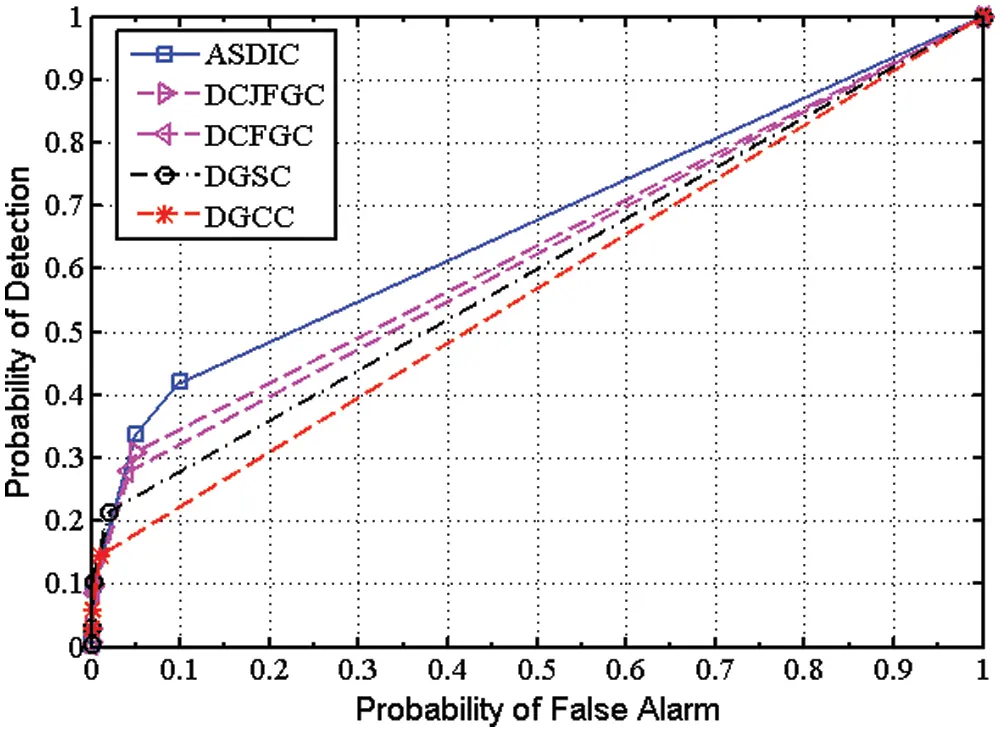Figure 10: The comparison of PFA verses probability of detection with existing optimized clustering approaches

The detection of performance is obtained by assuming SNR values which varies in the range of 0 to 30 dB and 0.1 is represented as the false alarm probability. As the SNR value increases, the probability detection will also increase linearly and reaches to fixes value of ‘1’. Fig. 11 shows the performance of detection with different value of SNR. The higher detection probability corresponds to lesser interferences with the PUs. In ASDIC, the PD is obtained as 0.937 better when compared with existing DSAC that obtained PD as 0.677 at 0 dB of SNR. The ASDIC curve converges faster to ‘1’ when SNR is higher than 4 dB as compared with existing optimized clustering approaches.Figure 11: The comparison of SNR verse PD with optimized clustering from the existing techniques

Fig. 12 describes the performance missed for detection of different PFA values with respect to ROC. The rate of PFA increases the Missed detection rate probability also reduces gradually for the ROC curve. The PUs will be active but will be not having the ability for identifying the primary transmission for the signal. As the rate of PFA increases the missed detection reduces the rate probability for the ROC curve. The effects of both the interferences among the SUs and PUs are caused. The existing DSA model has obtained PMD value reached up to 1 from 0.6 PFA value and linear graph obtained when moved to the point 1. The proposed ASDIC obtained PMD as 1 when the value of PFA was 0.1 and as the PMD reduces upto 0, the PFA will reach up to 1. The ASDIC obtains the performance well when identifying the primary transmissions for the false alarm rate highest when compared to the existing optimization process (e.g., PD = 0.372, PFA = 0.7, and PMD = 0.628) .

The ASDIC technique is the effective compared with the five existing optimized clustering methods which is used to save the transmission power with the shorter distances and to achieve the energy efficiency of clusters while restricting the interferences of primary user.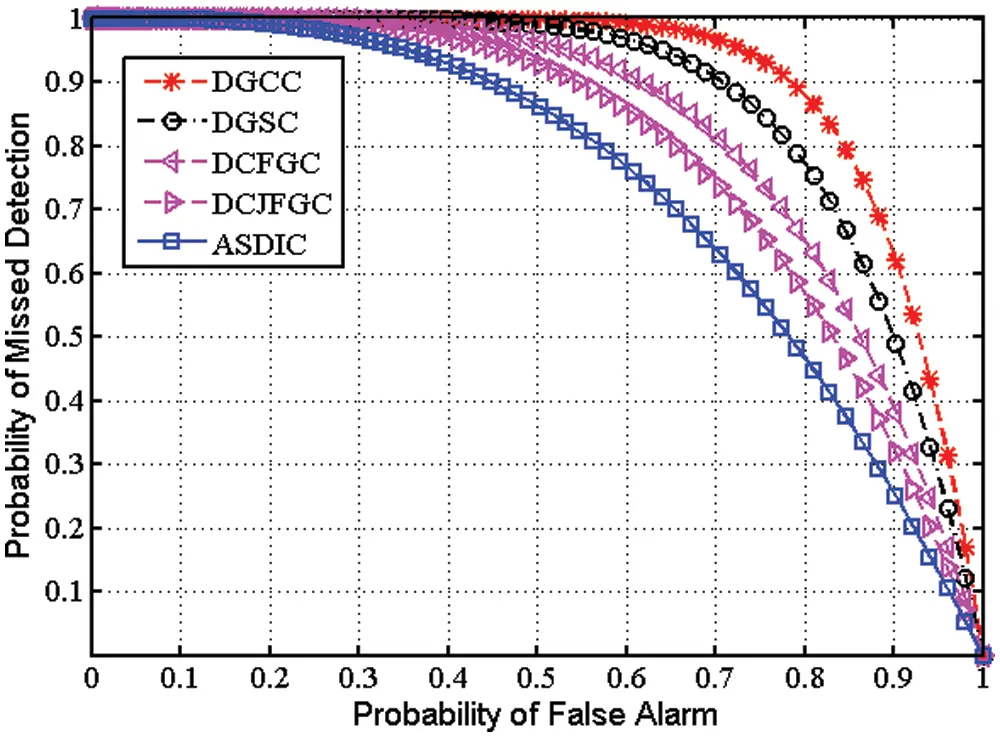Figure 12: The comparison of PFA verses missed detection probability with existing optimized clustering approaches

5  Conclusion

The performance from simulation shows the effective constancy and scalability for ASDIC. The analysis of performance shows 89.440% reduction in terms of power when compared to the existing models. The proposed ASDIC showed better CHs using the CRSN nodes with size 280 for 32.09 s convergence speed. The ASDIC showed effective performance of 9.646% when compared to DSAC for average node power from PUs effectively obtained 24.231% when compared to DSAC for average node power of SUs. The re-clustering process is performed that obtains steady stable showed lesser control within minimum average node power for lesser number of clusters.

The simulations results explain how the ASDIC algorithm is optimal for power saving and effective for detecting the spectrum of white spaces accurately. From the simulation, the ASDIC is giving lesser PFA with the higher probability of reducing sensing errors and detection as compared with the optimized clustering techniques. In the future work, the Convolutional Neural-Network (CNN) will be utilized for better clustering.

Acknowledgement: Thanks to the almighty.

Funding Statement: The authors received no specific funding for this study.

Conflicts of Interest: The authors declare that they have no conflicts of interest to report regarding the present study.

## References

1. R. Krishnan, R. Ganesh Babu, S. Kaviya, N. Pragadeesh Kumar, C. Rahul et al., “Software defined radio (SDR) foundations, technology tradeoffs: A survey,” in IEEE Int. Conf. on Power, Control, Signals & Instrumentation Engineering, Chennai, India, pp. 2677–2682, 2017.
2. R. Ganesh Babu and V. Amudha, “A survey on artificial intelligence techniques in cognitive radio networks,” in First Int. Conf. on Emerging Technologies in Data Mining and Information Security in Association with Springer Advances in Intelligent Systems and Computing Series, Kolkata, India, pp. 99–110, 2019.
3. M. H. Rehmani and R. Dhaou, Cognitive Radio, Mobile Communications and Wireless Networks. Cham: EAI/Springer Innovations in Communication and Computing, Springer International Publishing, 2019.
4. Y. Bin Zikria, F. Ishmanov, M. K. Afzal, S. W. Kim, S. Y. Nam et al., “Opportunistic channel selection MAC protocol for cognitive radio ad hoc sensor networks in the internet of things,” Sustainable Computing, Informatics and Systems, vol. 18, no. 1, pp. 112–120, 2018.
5. S. Wierzchon and M. Kłopotek, Modern Algorithms of Cluster Analysis. vol. 34. Cham: Springer International Publishing, 2018.
6. H. Zhang, Z. Zhang, H. Dai, R. Yin and X. Chen, “Distributed spectrum-aware clustering in cognitive radio sensor networks,” in IEEE Global Telecommunications Conf., Houston, TX, USA, pp. 1–6, 2020.
7. H. Wang, W. Wang, L. Cui, H. Sun, J. Zhao et al., “A hybrid multi-objective firefly algorithm for big data optimization,” Applied Soft Computing, vol. 69, no. 1, pp. 806–815, 2018.
8. M. Bidar and H. Rashidy Kanan, “Jumper firefly algorithm,” in Int. Conf. on Computer and Knowledge Engineering, Mashhad, Iran, pp. 267–271, 2013.
9. R. Ganesh Babu, M. S. Obaidat, V. Amudha, R. Manoharan and R. Sitharthan, “Comparative analysis of distributive linear and non-linear optimised spectrum sensing clustering techniques in cognitive radio network systems,” IET Networks, vol. 10, no. 5, pp. 253–263, 2021.
10. R. Ganesh Babu and V. Amudha, “Comparative analysis of distributive optimized clustering techniques in cognitive radio networks,” International Journal of Engineering and Technology, vol. 7, no. 3.27, pp. 504–507, 2018.
11. M. Rahim, A. S. Alfakeeh, R. Hussain, M. A. Javed, A. Shakeel et al., “Efficient channel allocation using matching theory for QoS provisioning in cognitive radio networks,” Sensors, vol. 20, no. 7, pp. 1872–1881, 2020.
12. B. Han, Y. Luo, M. Zeng and H. Jiang, “Spectrum-efficient resource allocation in multi-radio multi-hop cognitive radio networks,” Sensors, vol. 19, no. 20, pp. 4493–4502, 2019.
13. A. Shakeel, R. Hussain, A. Iqbal, I. L. Khan, Q. U. Hasan et al., “Analysis of efficient spectrum handoff in a multi-class hybrid spectrum access cognitive radio network using Markov modelling,” Sensors, vol. 19, no. 19, pp. 4120–4132, 2019.
14. Y. Fang, L. Li, Y. Li, H. Peng and Y. Yang, “Low energy consumption compressed spectrum sensing based on channel energy reconstruction in cognitive radio network,” Sensors, vol. 20, no. 5, pp. 1264–1272, 2020.
15. Y. Mi, G. Lu, Y. Li and Z. Bao, “A novel semi-soft decision scheme for cooperative spectrum sensing in cognitive radio networks,” Sensors, vol. 19, no. 11, pp. 2522–2531, 2019.
16. S. Wierzchon and M. Kłopotek, “Modern algorithms of cluster analysis,” in Studies in Big Data, 1st ed., vol. 1, Springer International Publishing, Springer, Cham, 2018.
17. T. Issariyakul and E. Hossain, Introduction to Network Simulator NS2, 2nd ed., Boston, MA: Springer US, 2009.
18. B. Abolhassani Hosseini and A. Hosseini, “Secure cognitive radio communication for internet-of-things: Anti-PUE attack based on graph theory,” Journal of Computer and Communications, vol. 5, no. 11, pp. 27–39, 2017.
19. S. Lavanya, B. Sindhuja and M. A. Bhagyaveni, “Implementation of an adaptive spectrum sensing technique in cognitive radio networks,” in Int. Conf. on Computing and Communications Technologies, Chennai, India, pp. 344–349, 2015.
20. W. Ejaz, G. A. Shah, N. Hasan and H. S. Kim, “Energy and throughput efficient cooperative spectrum sensing in cognitive radio sensor networks,” Transactions on Emerging Telecommunications Technologies, vol. 26, no. 7, pp. 1019–1030, 2015.This work is licensed under a Creative Commons Attribution 4.0 International License, which permits unrestricted use, distribution, and reproduction in any medium, provided the original work is properly cited.